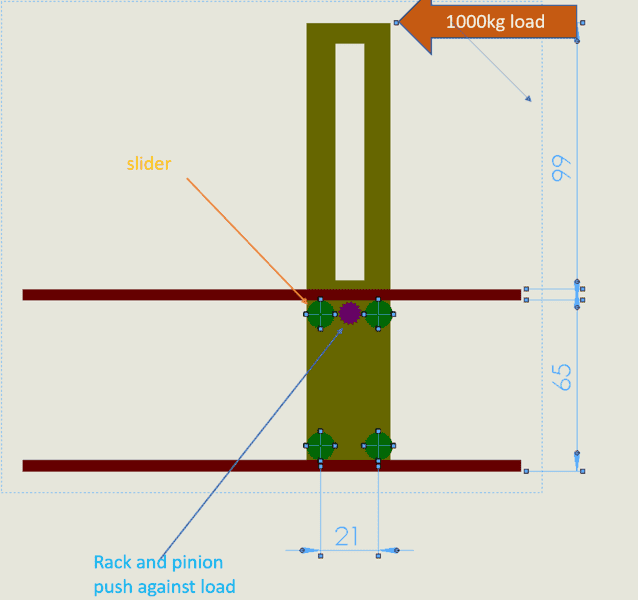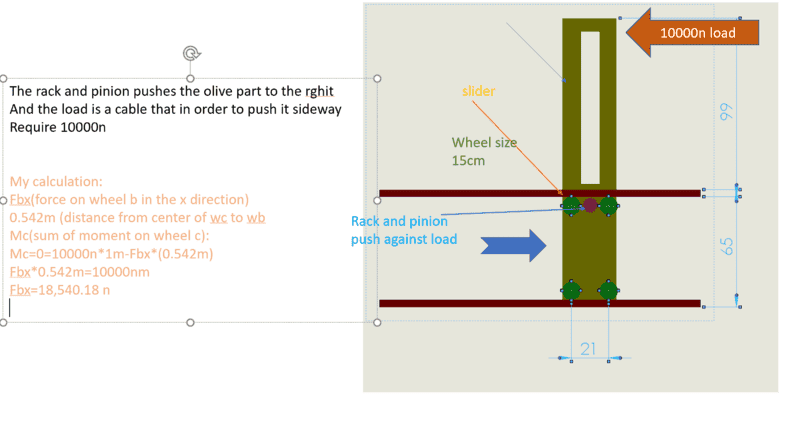# How to calculate the force on sliders?

• alik braz
In summary, the conversation is about a level wind system for a winch with a 1000kg load being applied to the top slider. The load is distributed to the bottom sliders through the use of four green wheels, and calculations need to be done to determine the forces on the wheels and the impact of the rack and pinion system. The conversation also discusses the use of statics to further analyze and calculate the forces involved.

#### alik braz

hello I am trying to calculate the force applied on the sliders in that sketch
it is a level wind system for winch
I am not an engineer but as much as i understend
the 1000kg load is 10000kn becose it is 1m from the top slider
the sliders at te top are 20 cm apert so the load will be 50000 kn on the slider if it was only slider at the top
but i don't know how the load ditrubot to the bottom sliders and betwen them
ill be very thenkful if somone can explain it to me in simpel way or add link to videos in that sobject
thanks#### Attachments

It's a little unclear how that load is being applied. 1000Kg represents a mass, which would normally present a downward force. But you have it in an arrow pointing to the left.
So one of these things are happening:
The olive colored part of the slider is being accelerate to the right of left while carrying that load. But you have not specified any such acceleration or movement.
Either left is the downward direction, or force of a 1000Kg mass in 1G of gravity is being applied to the top of the slider in the direction indicated.

You need to tell us which. In the meantime, I will assume the latter: 10,000KN of force is applied in direction indicated by the arrow.

At this point you will need to label your parts. I am going to call the four green wheel Wa and Wb (incontact with the top slide bar and the sprocket) and Wc and Wd (in contact with the lower slide bar) - with Wa and Wc on the left and Wb and Wd on the right.

Let the horizontal axis be the x-axis and vertical the y-axis. So your 10MN force is (-10,0)MN.
The olive colored bar is resisting this force through its contact with Wb (which can apply force in both x and y) and Wc (which can apply force along the y-axis only).

But to continue the analysis, you will need to specify:
the thickness of the top brown slide bar
the diameter of the green wheels.

----
Also: At this point you need to start showing us some more of your own effort on analyzing this.

Last edited:
•berkeman
Looking more carefully at your comments, it appears that the thickness of those brown bars must be 1cm.
It also appears that you need to know two things:
1) The forces on Wa, Wb, Wc, and Wd when the only force being applied is through gravity applied to the load.
2) The forces on Wa, Wb, Wc, and Wd when the only force being applied is through the purple sprocket and the inertia of the load.

The wheel size is still critical. Looks to be about 12cm.for the calculation the distance from the center of wb to the top of the olive part is 1m
I add the size of the wheels
and the force is a result of the rack and pinion pushing against a cable that blocks the olive part in the right side
i don't know how to take in consideration the force of rack and pinion (i have to add it again or not)
and i am not sure that i am in the right direction at all with that calculation
thank you very much
alik

#### Attachments

•jim mcnamara
Thank you so much
Can you please tell me what should I search for to learn more about it and to know how to calculate things like this?
Statics?
Any specific subject?
Thank you again
Alik

•jrmichler
Use search term statics. One of the first hits is https://www.engineer4free.com/statics.html. I took a quick look at that site, and it looks pretty good. They do state that: The lessons build on each other so we recommend that you start at the top of the list and watch them all in order. They are not kidding. You need to start at the beginning, and watch each video in order. By the time you finish, you will easily be able to find the forces on your mechanism.

Thank you very much

## 1. What is the formula for calculating the force on sliders?

The formula for calculating the force on sliders is F = m x a, where F is the force in Newtons, m is the mass of the slider in kilograms, and a is the acceleration in meters per second squared.

## 2. How do I determine the mass of the slider?

The mass of the slider can be determined by using a scale or balance to measure its weight in kilograms. If the weight is not given in kilograms, it can be converted using the formula 1 kg = 9.8 N.

## 3. What is the acceleration for calculating the force on sliders?

The acceleration for calculating the force on sliders depends on the type of motion. If the slider is moving at a constant speed, the acceleration is 0. If the slider is accelerating, the acceleration can be calculated using the formula a = (vf - vi)/t, where vf is the final velocity, vi is the initial velocity, and t is the time in seconds.

## 4. Can the force on sliders be negative?

Yes, the force on sliders can be negative. This means that the force is acting in the opposite direction of the motion. For example, if a slider is moving to the right and experiences a negative force, it will slow down or move to the left.

## 5. How can I apply this calculation to real-life situations?

The calculation for force on sliders can be applied to various real-life situations, such as calculating the force required to push a shopping cart or determining the force exerted on a car during a collision. It is a fundamental concept in physics and can be used in many different scenarios to understand and predict the motion of objects.